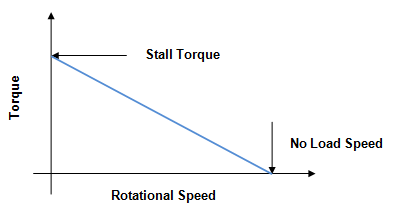# Relation between Torque and Speed

## Introduction

There relation of torque with the speed of an object is an important part of physics as it is integrally connected to the motion and rotating capability. The relation between these two components is integrally and very much connected to the power of the object. The value of torque is often extracted by “Power/ Speed.” This statement is the most basic and necessary information to know about the relation between speed and torque.

## What is Torque?

Torque is a significant product of the radius of rotation and force. As a result, in the case of an increasing radius, the torque is also found to increase (Lenzo et al. 2018). This finally results in torque. Torque is also known as the rotational equivalence of an object’s linear force.

Images Coming soon

Torque is a measure of force that is the reason behind leading an object to rotate. Angular acceleration is also found to be a result of torque. Description of torque also includes a moment of force (Okoroha et al. 2019).

The lever arm and moment arm are the two most important terms that are connected to the relation of torque and speed. Torque is presented by the symbol ‘T’. Si unit of Torque is N.m (Newton Meter). Dimension and SI base units are $\mathrm{ML^{2}T^{-2}}$ and kg.$\mathrm{m^{2}.s^{-2}}$. There are main two types of torque in physics and these are dynamic and static torque. Static torque is found to produce any kind of angular acceleration. As an example, cycling can be mentioned in which no such kind of acceleration is found.

## What is Speed?

The speed of an object is used to measure the total distance that is covered within a particular unit of time. The speed of an object is considered a scalar unit of quantity in physics. In the time of determining the speed of an object, there is no need to establish the proper direction of an object’s movement (Andrade et al. 2020). The term and meaning of velocity possess a very significant difference. Velocity is a vector quality. It can also be described as the speed of an object that is moving in a particular direction.

The measurement of an object’s speed is presented by both CGS and SI units. In CGS mode, the speed is presented with cm.s-1 and in SI mode, the unit becomes $\mathrm{ms^{-1}}$(Osawa et al. 2019). There are four significant types of speed and these are instantaneous, average, uniform, and variable speed.

## Relationship between Torque and Speed

There is an integral relation between torque and speed based on which the differences in motion of an object can be determined. The relation between speed and torque is significantly proportional to each other. In the case of presenting the torque of a rotating object, the ratio between angular velocity and power is important.

In the case of a rotational motion, the torque is often expressed as the multiplication of force value with radius value (Sun et al. 2019). As a result, the value of force is the value in which torque is divided by the value of radius. The power value can be calculated by multiplying linear distance and force and then dividing the result by time. The multiplication of torque and angular velocity extracts the value of power.

Images Coming soon

## Relation between Speed and Torque: Derivation

Being a rotatory motion, it s very easy to derive the significant and unique relation between torque with power. Comparison of linear equivalent is important for this derivation. The covered angle is to be multiplied by a radius of movement for extracting the value of linear displacement. The circumference of a wheel is integrally connected to the linear displacement of an object (Artetxe et al. 2018). The formula of Linear distance is “Linear distance= Radius* Angular velocity* time”. As per this related mathematical formula, force is inversely and significantly proportional to the value of speed, also known as angular velocity.## The difference between Torque and Speed

Torque Speed
It is related to rotational force. It is related to the rotation rate of an object.
The output of torque helps an object to increase its speed. Speed can be calculated by considering (Revolutions per minute) RPMs.

## Conclusion

The calculation of Torque is done by following a formula in which torque is equal to the result in which the power of the object is divided by that object’s speed. The value of power is a relevant part, based on which the torque of an object can be calculated. Comparison of the linear equivalent is also a mentionable part. In the equation of torque and speed, the value of velocity and speed are interchangeable in nature. The most important reason behind this is the torque that always possesses the speed in a stable, particular, and confirms direction.

## FAQs

Q.1. What is meant by linear displacement?

Ans. Linear displacement is the particular amount of distance that is covered by an object within a specific time. The circumference of an object’s rotation is also related to the concept of linear displacement. The value of a product’s angle with radius is also related to the concept of linear displacement.

Q.2. What is defined by angular speed?

Ans. Angular speed is used to define the speed at which a central angle of a rotating body is found to change in respect of different times. Angular speed is expressed by “$\mathrm{\omega =\theta t}$”.

Q.3. What are the two most important components with which the rotational motion can be characterized?

Ans. The value of speed and torque are the two most important components with which the value of rotational motion is characterized. Rotational motion is also related to the power which is acquired by multiplying speed and torque.

Q.4.What is the relation between torque and output speed?

Ans. An incensement in torque results in decreasing value of output speed. These two components are related inversely.